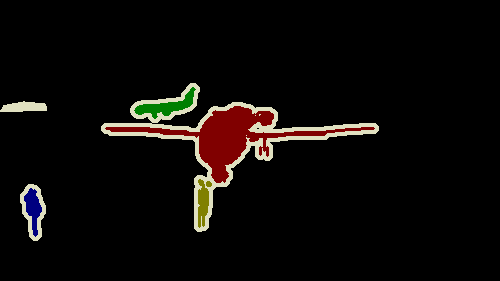# Image lost its pixels(color) after reading from PIL and converting back

Data Fatching

``````import PIL.Image as Image
import os
import torch
images=torch.zeros(len(df),3,128,128)
for i in range(len(df)):
ak=Image.open(("/content/VOC2012/SegmentationObject")+"/"+df['a'][i]+".png")
ak=transform(ak)
images[i]=ak
``````

Transform–

``````from torchvision import transforms
transform=transforms.Compose([
transforms.CenterCrop((128,128)),
transforms.ToTensor(),
])
``````

Back to PIL Code-

``````to_pil = torchvision.transforms.ToPILImage()
tar = to_pil(img)
``````

tar is(Image after converting back to PIL(above code))–original image-Is this because of CenterCROP in the transform.
Or what is the problem.
cv2(Open CV) help me and no color loss happens in cv2.

In the docs it says that you can give a mode to the ToPILImage(). Try `ToPILImage('RGB')`

If that doesn’t work. Can you print the size() of your tensor before you transform it to a PIL image?

Could you also print the mode of your input image. In the for loop before the transform do a `print(ak)`

1 Like

No, `ToPILImage('RGB')`– this did not work.

Size of my tensor–
`torch.Size([3, 128, 128])`

`Could you also print the mode of your input image. In the for loop before the transform do a print(ak)`

What here you are asking??. I am not getting the meaning of
`mode of your input image`

``````import PIL.Image as Image
import os
import torch
images=torch.zeros(len(df),3,128,128)
for i in range(len(df)):
ak=Image.open(("/content/VOC2012/SegmentationObject")+"/"+df['a'][i]+".png")
print(ak) # This will tell you information about the image such as mode and size
ak=transform(ak)
images[i]=ak
``````
1 Like

This code produces the output `<PIL.Image.Image image mode=RGB size=128x128 at 0x11A40AEF0>`. I’m guessing it has to do with the input data being in a non-standard way. What dataset are you working with?

``````from PIL import Image
import torch
from torchvision import transforms

transform=transforms.Compose([
transforms.CenterCrop((128,128)),
transforms.ToTensor(),
])

images=torch.zeros(2,3,128,128)
for i in range(2):
ak=Image.open('testimage.jpg') # Mode=RGB=3 channels
ak=transform(ak)
images[i]=ak

to_pil = transforms.ToPILImage()
tar = to_pil(images)
print(tar)

``````

Edit: I tried the image you uploaded and it isn’t a normal RGB image. Says `<PIL.PngImagePlugin.PngImageFile image mode=P size=500x281 at 0x11231B1D0>`. You can read at the different modes at the docs

1 Like

I am working with pascal-voc-2012

`https://www.kaggle.com/huanghanchina/pascal-voc-2012`

Cool. Do you understand what the problem was and think you can continue from here :)?

1 Like

Yeh I got the problem and I have to handle the image by some other way suggested by the doc link.
Thnx

I tried using different modes given in the docs but cannot able to resolve the problem. The mode for the image is ‘P’. And after using transform my image has shape `([1,128,128])`, so basically the problem occurs in transform as transform converted it from `RGB to Binary`

Hmm, lets see if we can figure this out together.

The input image has the mode ‘P’ as you say. This is a 1-channel image which maps to another colorspace and is represented by a uint8. This means that it can show a maximum of 256 colors. This is in fact very much the mode ‘L’ (gray image) which also is a 1-channel image represented by an uint8.

The difference here is that a gray image can show 256 shades of gray, ranging from black to white. The image you are working with can represent 256 colors (blue, green, yellow, etc) but never any mix of these colors. There aren’t any shades in between.

You will never get a normal color-image from the image you are working with.

Now, that we know what kind of data we are working with, what is the intended use of the data? I’m guessing you have a corresponding image, like a .jpg, that you use as input and this data as ground truth.

I wrote this little script that converts an image from PIL image mode P, to tensor and back. Just fill in one of the paths and it should show you a color image. Just note that I don’t know how this works if you have multiple images with different colors

``````from PIL import Image
from torchvision import transforms

im = Image.open('PATHFORIMAGE.png')
palette = im.getpalette()

transform = transforms.Compose([
transforms.CenterCrop((128,128)),
transforms.ToTensor(),
])

tr_im = transform(im)
to_pil = transforms.ToPILImage()
back_im = to_pil(tr_im)

p_im = back_im.convert('P')
p_im.putpalette(palette)
p_im.show()
``````

Outputs ->2 Likes

Thnx a lot…now with this post I actually understand the problem. And thnx for different shades explain(it made me easy to catch).

1 Like

One more thing. I have to pass color palette to it. So during the conversion is my tensor lost its information of color palette or colors ?

Yes that info is lost in the tensor. If you want the original colors used you have to save the palette.

If you are ok with the red being for example green and the yellow being blue, you can use a new palette.

1 Like

So Is their any function to retain those colors(pixels not to get modified) . I have to later use the image as Target in my model to compute lost. So missing pixels can cause problem.